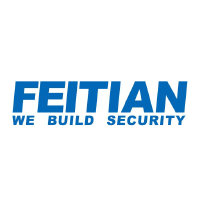# Feitian Technologies Co Ltd SZSE:300386Feitian Technologies Co Ltd
SZSE:300386
Price: 10.11 CNY +0.4%
Updated:

## DCF Value

This DCF valuation model was created byAlpha Spread and was last updated on Jan 29, 2023.

Estimated DCF Value of one300386 stock is 3.2 CNY. Compared to the current market price of 10.11 CNY, the stock is Overvalued by 68%.

DCF Valuation FAQ:
DCF Value
Base Case
3.2 CNY
Overvaluation 68%
DCF Value
Price3.2
DCF Value
Feitian Technologies Co Ltd Competitors:
DCF Valuation
300868
Shenzhen Jame Technology Corp Ltd
300689
Shenzhen Chengtian Weiye Technology Co Ltd
CNC
Concurrent Technologies PLC
000977
Inspur Electronic Information Industry Co Ltd

## Present Value Calculation

The first step in the DCF calculation process is to estimate the company's future free cash flow. Once free cash flow is forecasted, it is discounted at a risk-appropriate discount rate. The resulting value is the present value of the company's free cash flow. You can change any model inputs below.

Available Valuation Models
1 valuation model availableUpdated on Jan 29, 2023
Default Valuation
Other investors haven't shared any valuation models for Feitian Technologies Co Ltd.
Model Settings
Operating Model
Discount Rate
8.51%
Forecast Period
5 Years
Terminal Growth
0%
Discount Rate
8.51%
Terminal Growth
0%
Growth Period
5 Years

### DCF Model Base Case ScenarioDCF Model
Currency: CNY
Millions

To view the process of deriving the DCF Value of one share from the estimated Present Value, see the DCF Value Calculation block.

## DCF Value Calculation

Depending on which type of operating model is chosen (equity or whole firm valuation model), the resulting value is either the value of equity or the value of the entire firm. In the case of the latter, to move from the value of the firm to the value of equity, liabilities are subtracted and assets are added.

### Capital Structure From Present Value to DCF ValuePresent Value 602M CNY + Cash & Equivalents 716M CNY + Investments 54.5M CNY Firm Value 1.4B CNY - Debt 17.6M CNY - Minority Interest 18.3M CNY Equity Value 1.3B CNY / Shares Outstanding 418M 300386 DCF Value 3.2 CNY
Overvalued by 68%

To view the process of calculating the Present Value of Feitian Technologies Co Ltd' future free cash flow, see the Present Value Calculation block.

## Valuation Analysis

After the valuation process, in order to gain a deeper understanding of the relationship between risk and opportunity, it is useful to study the dynamic of company's valuation under various inputs to the DCF model in use.

Revenue
978M 1B
Operating Income
41.7M 67.1M
FCFF
36.1M 55.1M

### Sensitivity Analysis DCF Value Sensitivity AnalysisEstimated DCF Value of one300386 stock is 3.2 CNY. Compared to the current market price of 10.11 CNY, the stock is Overvalued by 68%.
1. Present Value Calculation. Using the DCF Operating Model we projectFeitian Technologies Co Ltd's future free cash flow and discount it at a selected discount rate to calculate its Present Value (602M CNY).
2. DCF Value Calculation. We use the company's capital structure to calculate the total Equity Value based on the previously computed Present Value of the free cash flow. Dividing the Equity Value by the number of shares outstanding gives us the DCF Value of 3.2 CNY per one300386 share.